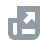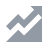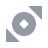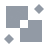Pay with
Markets
Derivatives
Earn
NFT
Institutional
Feed
USD

# FAQAccount FunctionsTutorialBinance Fan TokenBinance EarnCrypto Deposit/WithdrawalCrypto DerivativesFinanceAPISecurityOther TopicsBinance ConvertNFTVIP
Home
Support Center
FAQ

# Binance Cross Margin Trading Guide

2020-03-26 08:59

## Margin Service Terms

By using the Margin Services, you acknowledge that you have read, understood, and accepted all of the terms and conditions in the Margin Service Terms, and you acknowledge and agree that you will be bound by and will comply with the Margin Service Terms. This Cross Margin Trading Guide does not govern the Margin Services and is provided for your information and convenience only. In the event of any conflict between this Cross Margin Trading Guide and the Margin Service Terms, the Margin Service Terms shall prevail.

## Collateral Assets

You can use your Cross Margin account assets as collateral assets for Cross Margin trading. For more details, please refer to the Margin Data page.

## Interest

Simple interest accrues on an hourly basis. Interest is calculated first at the time of the successful advance of the relevant Margin Loan, which will be counted as the first hour. It will be calculated again at the next full hour (which will be counted as the second hour), and then every following full hour until the Margin Loan is repaid. This means that even if the Margin Loan is outstanding for less than an hour, interest will be charged for one full hour.
Interest is calculated according to the following formula:
Interest = Principal Amount Outstanding * (Hourly Interest) * Number of Hours the Loan Has Been Outstanding
Users may repay their debts at any time. Repayment shall be deemed payment of interest first, and after the interest is fully paid, repayment of the principal of the relevant Margin Loan.
Outstanding loan interest shall be included in the calculation of the Margin Level. Assuming no payments of interest are made for an extended period, the Margin Level of the user's Cross Margin account may deteriorate, leading to the risk of a margin call or even forced liquidation.
Outstanding loan interest is included in the Margin Level calculation. Assuming no interest payments are made for an extended period, the Margin Level of your Cross Margin account may deteriorate, leading to the risk of a margin call or even forced liquidation.
Margin account interest rates are adjusted occasionally to reflect market conditions. You can find the latest interest rate on the Margin Data page.

## Margin Level and Risk Controls

You may only use the assets in your Binance Cross Margin account as collateral for Margin Loans. However, the digital assets in your other accounts are not included in the Margin calculation for Cross Margin trading. The table below illustrates the risk controls at different margin levels.
The Margin Level of a Cross Margin Account is calculated as follows:
Margin Level = Total Asset Value of a Cross Margin Account / (Total Liabilities + Outstanding Interest)
where:
Total Asset Value of a Cross Margin Account = Current Total Market Value of All Digital Assets in the Cross Margin Account
Total Liabilities = Current Total Market Value of All Outstanding Margin Loans in the Cross Margin Account
Outstanding Interest = Principal Amount Outstanding * Number of Hours the Loan Has Been Outstanding * Hourly Interest Rate - Interest Paid

## Collateral Value and Collateral Value Ratio

Collateral Value refers to the total value of all assets in your Cross Margin account in USDT. It also takes into consideration the relevant Collateral Ratio – the percentage at which the relevant asset is valued. For more details on the Collateral Ratio for each digital asset, please refer to the Margin Data page and Binance Cross Margin Collateral Haircuts article.
The Collateral Value Ratio will determine the maximum amount you can withdraw from your Cross Margin account. When your Collateral Value Ratio is > 2, you can transfer excess assets from your Margin Wallet to your Spot Wallet, only if the Collateral Value Ratio remains above 2 after the transfer.
The Collateral Value Ratio of the Cross Margin Account = Collateral Value / (Total Liabilities + Outstanding Interest), where:
Collateral Value = Current Total Market Value of All Digital Assets in the Cross Margin Account * Collateral Ratio (calculated separately for each asset and then aggregated)
Total Liabilities = Current Total Market Value of All Outstanding Margin Loans in the Cross Margin Account
Outstanding Interest = Amount of Each Margin Loan * Loan Time (in hours, at the time of the calculation) * Hourly Interest Rate - Interest Paid

## Sub-accounts

A master account can only have 10 sub-accounts in Margin trading. The maximum loan limit of a sub-account is 1/10 of the loan limit of the master account.